# Operating Systems - Main Memory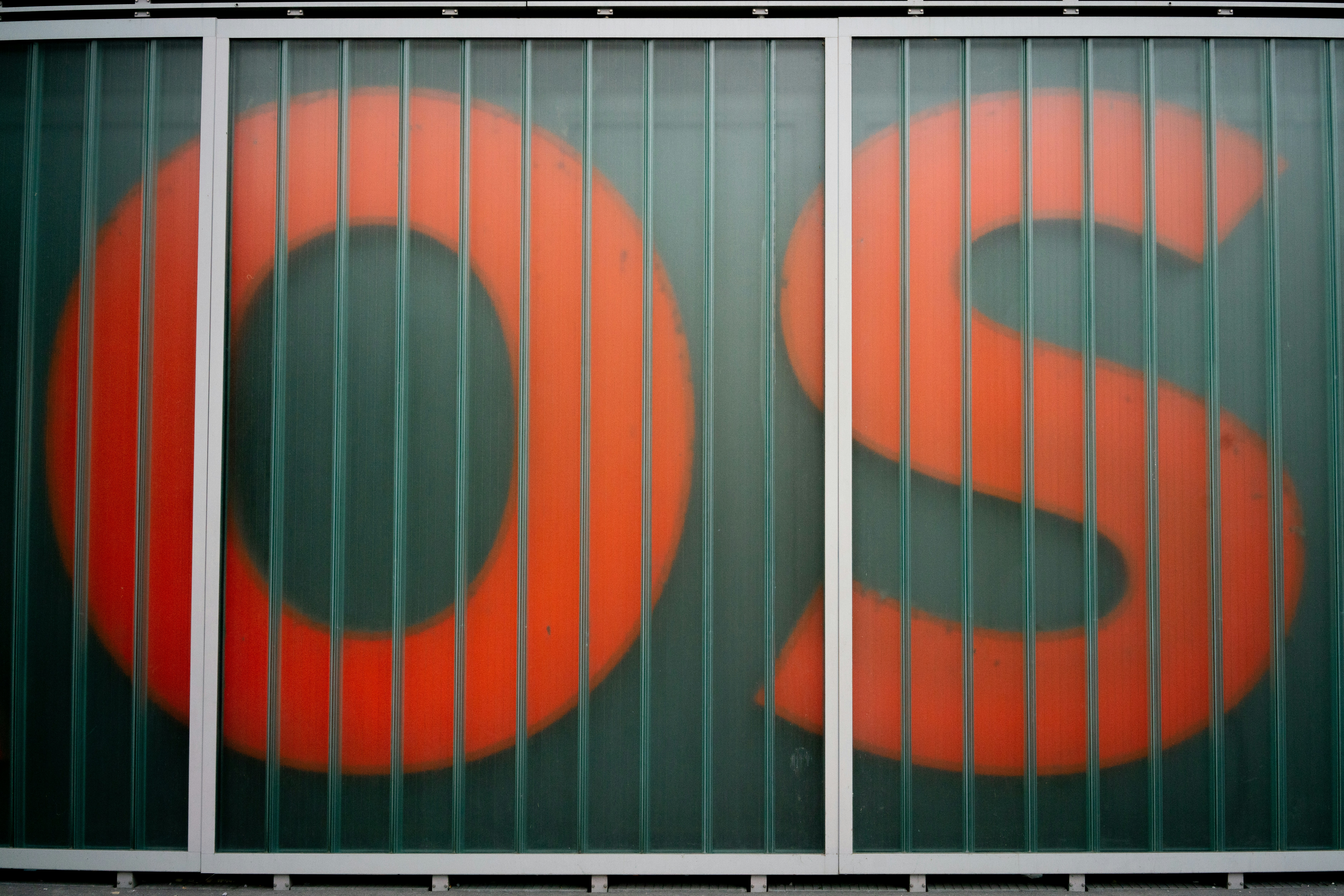There are various ways to manage memory. The strategies vary from a primitive bare-machine approach to paging and segmentation. Each has its own advantages and disadvantages. We begin by looking at some hardware.

## Hardware

### Basic hardware

The figure below illustrates physical memory, with the operating system along with some processes loaded into it. The numbers refers to addresses. Since every address points to a cell in memory, and a cell consists of a byte (8 bits), the numbers are in bytes. In the picture we therefore have a total of 1024 KB, which is pretty small for a physical memory.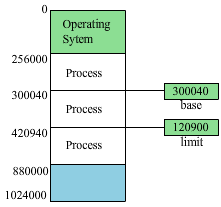Figure 1: Memory layout with base and limit for logical address space

We can see that the operating system is placed first, at memory cell 0. There is nothing preventing us from placing the operating system anywhere else in memory, but it's often the case that it's placed at the first address. After the operating system, we have a couple of processes. Processes have a base and a limit. The base is just the address where the process is starting at. The limit is the amount of cells that a process is using. To find the last address of a process, we use the formula base + limit - 1. Notice the "- 1" in there. If we would just calculate base + limit, the result would be the first address of another process. This will become clear if you look at the picture above. Base (300040) + limit (120900) = 420940, which is the first cell for the third process.

Now, what if a process tries to access another process memory space? A process should only be able to access its own memory space. There's a piece of hardware that makes sure this doesn't happen, called memory management unit (MMU). The MMU can operate in different ways depending on what strategy we use, the figure below illustrates our situation.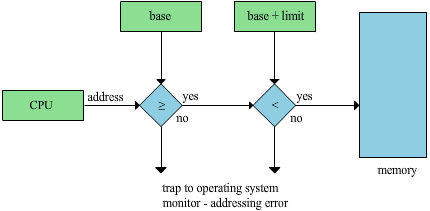Figure 2: A schematic of hardware protection for base and limit memory protection.

Imagine if the CPU is working with a process, and that process wants to get something from a memory cell. The CPU will generate this address and send it out on the address bus, but before it gets to the memory, as the figure suggests, the signal will go through some tests. These tests are done by the MMU. It first checks if the address is greater than or equal to the base. If this condition is true, it proceeds by checking if the address is less than base + limit. If this condition is true as well, the process can access the desired memory cell. If one of the conditions is not true however, it will tell the operating system that something is wrong, and the operating system will deal with the problem.

### Binding memory in a process

When you create a program, you begin with writing the source code. To execute your program and make it a process, the source code has to be compiled, linked, loaded and executed. I actually wrote an article about these steps in another article, C++ Creating a program, behind the scenes.

When do we decide where the process should reside in memory? We have three options:

• Compile time. If we decide where the process should reside at compile time, absolute code can be generated. The code has to recompile if the starting location changes at some later time.

• Load time. If we don't know at compile time where the process will reside in memory, then the compiler must generate relocatable code. We then only need to reload the user code if the starting address changes at some later time. The final binding is delayed until load time.

• Execution time. If the process can be moved during its execution from one memory segment to another, then binding must be delayed until run time.

### Relocation registers

Let's add some flexibility here. Addresses generated by the CPU is referred to as logical addresses. Real addresses, i.e where the addresses actually exists in memory is referred to as physical addresses. All logical addresses starts at 0. This means that every process "think" that it has the base 0.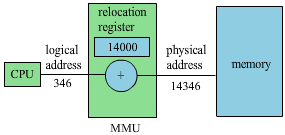Figure 3: An illustration of a relocation register

Figure 3 above shows us that the CPU generates a logical address, 346, which is then going through the MMU. The MMU adds this address with whatever value is necessary to translate it to its real physical address. This value is stored in the process PCB (Process Control Block). In this situation, all the MMU has to do is to check whether the logical address is less than our limit, which is the amount of cells our process possesses. This limit is also stored in the PCB. If our condition is true, we just add our relocation register, and we get our physical address (Figure 4).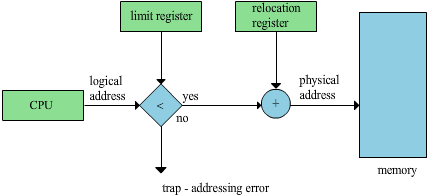Figure 4: A relocation register with base and limit properties

## Memory Allocation

### Contiguous allocation

Have a look at Figure 5. Imagine if there was a process above P1 at some time, executing som code until its done, then leaving us with a hole like the figure is showing us. Another process, P3, is ready and want to start executing after this. At a first glance, it looks like we have enough memory to load P3. But we can't place it in either of the holes, they are too small. The total amount of free space is enough for P3, but the memory has been fragmented. This is called external fragmentation. This problem gets worse and worse when processes comes and goes.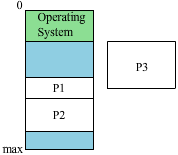Figure 5: External fragmentation

Let's forget P3 for a while, it won't work anyway. I'm not gonna provide a new image for this one but imagine that P4 is as small as the smallest hole (at the very bottom) and is ready to be executed. Obviously, this time it will work. Now the question is, where do we put it?

• First-fit. In this algorithm, a process will look for a space from top to bottom. If a place where it fits is found, the process will be put there. In Figure 5, our new process will be put just below the operating system.

• Best-fit. Here the process will first go through the whole memory space and compare them to see what position leaves as small holes as possible. Since we said that P4 is the size of the smallest hole in Figure 5, it will be a perfect fit there. But what if it was a little smaller? That would leave holes as well.

• Worst-fit. It sounds quite silly to put a process where it fits worst. This means that we will allocate the largest hole. Just as in best-fit, we need to search the entire list and compare the holes, but this time to see where the largest hole is. In Figure 5, this will put the process just below the operating system, like first-fit.

The most common strategies is first-fit and worst-fit. The next sections explains non-contiguous allocation, paging and segmentation.

### Paging

In paging, we're going to split the logical address into two parts. We call the parts page number and page offset (displacement). Pages are typically1 KiB in size. The size is a power of two, so if the page size is the last 10 bits, then each page will be  bytes (or 1 KiB) in size. The size of the page must be equal to the size of the physical counterpart, the frame. The frame size, (and hence the page size) are determined by hardware. Each page is mapped to a frame, and this mapping is kept in a page table. Figure 6 provides an overview of paging: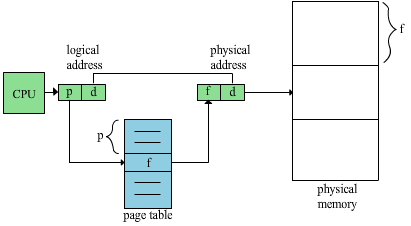Figure 6: An overview of paging

Pay attention to Figure 6. When the CPU generates a logical address, we no longer look at it as a simple number. We split it in two parts, the page number (p) and displacement (d). Following the page number, we will arrive at a page table (blue area in Figure 6). By using the page table, we can translate our logical address to a physical address, and thus get access to our frame. The displacement is just an offset, and will be the same after the lookup, so we just leave it as it is.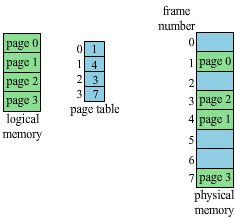Figure 7: A pagetable

Its the memory management units duty to make this work. In Figure 7, let's find page 0 in physical memory. If you look at the page table in the middle, you see that page 0 is in frame 1. Ok, so we (the MMU) look at the physical memory, in frame 1 we do in fact have page 0. With this setup, everything is quite scattered in the physical memory.

So what's the point of paging? We eliminate external fragmentation. Remember when we tried to fit P3 in Figure 5 but it just wouldn't work? Well, problem solved. Let's dig deeper.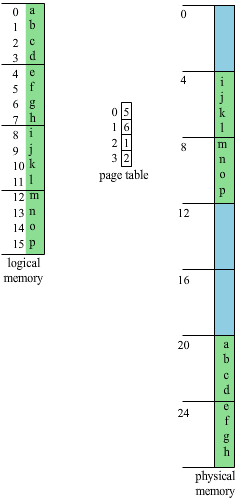Figure 8: Allocated memory using paging

It's not enough to just look at the pages, there's a displacement too. Let's say we want to know where the letter n is in physical memory. We can see that every page (and frame) have 4 bits each (which is extremely small, I said before that they typically consists of 1 KiB, but this is just for illustration). If we count the pages from top until we find the page with n, we'll see that n is on page 3. Remember that we start counting on 0, not 1. The offset is 1, it's on bit number 13. In the page table, we see that page 3 is in frame number 2. First letter in frame number 2 is m, adding our offset (+1) will get us to our desired location, and there we find our n.

The page table is usually stored by the OS in the process's PCB. The dispatcher loads the process's page table into the hardware, if the page table is sufficiently small. Bigger tables must be kept in the memory. A page table base register then points to the page table's position in memory. However, this requires a memory lookup, i.e. each memory reference requires two references. Luckily, we got a solution to this problem. The solution is to add a translation look-aside buffer (TLB), a very fast hardware cache.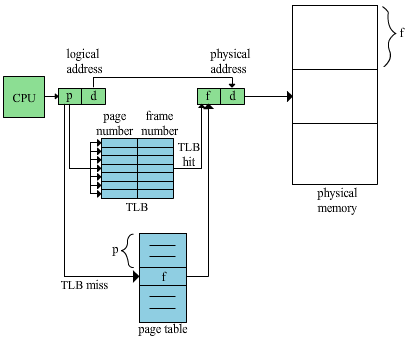Figure 9: Paging with a TLB

Figure 9 illustrates paging with a TLB. The idea is to store the most used pages at the moment. We can't store everything in the cache because the page table can get way too big. So the CPU generates a logical address, this time we check if the page number is in the TLB first. If it is, great, we got out frame and are good to go. If it's not there, we have to check the page table which takes a longer time.

Some TLBs have address-space identifications (ASIDs). The acronym is probably not what you read at the first time, but whatever, let's proceed. ASIDs allows the TLB to handle pages for different processes simultaneously. Hence we don't need to clear it every context switch.

We must have some protection for the pages of a process. What happens when the page table is larger than necessary for a specific process? Consider a system with a 14-bit address space, a process with the logical address range 0 to 10468, and a page size of 2 KiB: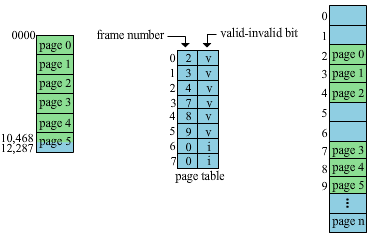Figure 10: Paging with valid-bits

A problem that arises with paging is internal fragmentation. In the example above, we only needed memory for up to about four and a half page. This leaves us with a half unused dead page. So when we solve the external fragmentation problem, we end up with internal fragmentation. This problem is extremely smaller than external fragmentation, so we're fine with it.

In Figure 10, I added a valid-invalid bit. This bit is just telling the system if the translation is valid or not. Using the same principle, we can add additional bits. For example, we can have bits for specifying whether reading, writing or execution is allowed on these pages. We can implement execution prevention for certain memory regions, e.g where the web browser stores data downloaded from the web. Several processes can share read-only pages, hence requiring less memory: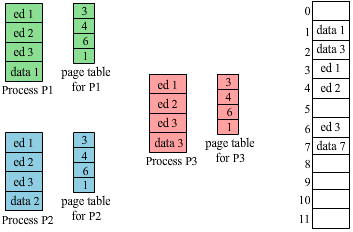Figure 11: Shared page example

Imagine that you just started up three processes of the same editor. A lot of the code for the editors are the same. All three processes have ed 1, ed 2 and ed 3 in common. Since they share the exact same code, we can map them to the same location in the physical memory by letting them share frames.

### Segmentation

In segmentation, we try to divide processes in segments in such a way that we as programmers are used to think in. Maybe the function sqrt is a segment, the stack is a segment etc.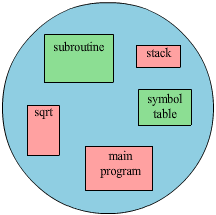Figure 12: Segments

If we only use segmentation, Figure 13 illustrates what the MMU could be working with. Now we have a base and a limit again. Instead of a page table we get a segment table. The CPU generates an address, then we check what segment it belongs to. What's the base and limit? After that, we do our test, is the address valid? If it's valid, we can continue and get our physical address.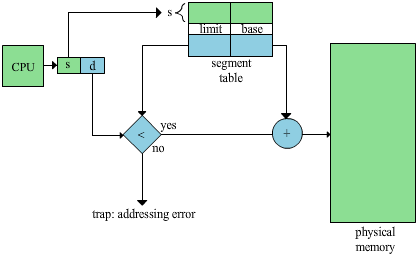Figure 13: Segmentation hardware

So segmentation is another way to try to avoid external fragmentation. It's not super effective, since segments can get rather big, but it helps a little. Segmentation are often combined with paging. Processes are first divided into segments, then they are divided into pages.